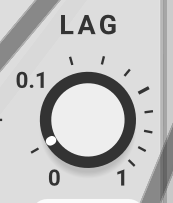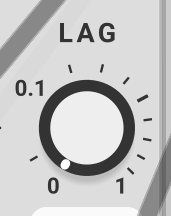# Looking for a compact CV to gate module

So practically this is what I’m after:

``````if CVinput>1.23 and CVinput<4.56 then
CVoutput=10
else
CVoutput=0
end if
``````

I’m looking for a compact solution. The smaller the better.

I’m already fairly good at making things the complicated way.I was in the same situation the other day. I ended up using a series of comparators. But I think this might work better

I haven’t tried it yet.

You’ll need two comparators, one for the lower voltage, one for the upper voltage and a logical AND to bring the “over” of the former and the “under” of the latter together as shown here.

The binary comparator compares two sets of 8 bit binary patterns so won’t be useful for this.

It may be simpler to use one of the modules that allows you to enter formulas.

3 Likes
3 Likes

The window comparator doesn’t quite work the same though.

1 Like

turn lag to 0 (?)

It is!

If you use Frank Buss Formula you can use this formula:

(w>1.23)*(w<4.56)*10.0

Turn off the clamp for 0-10V output.

1 Likeis 0.01is 0

OK champ.

1 Like

I think your solution is better - I had to calculate the mean between the two voltages for “B” and the diffence/2 for the window.

It’s still a great tip. I could never get bogaudio CMP to work properly, now I know why. Thanks.

1 Like

Thanks for all the replies! I keep on experimenting. CMP is clearly a interesting solution I haven’t though of.

I secretly hoped that there’s already some barebone module that I missed in Library. I.e.:

What does the parameter next to the CV input do?

An attenuator for the CV input. Looked like a good idea. (Not sure any longer.)

Nothing complicated about that - it is intuitive, compact, and kind to the CPU. I would be inclined to use AO-106 instead so as to use a single module and eliminate additional sample delays. But that does take more space.

Note that if your patch happens to have a constant 10V CV source available, then you can eliminate the last step by patching 10V into step 2 Y and changing the formula to “if X>C → Y/0”

Note also that of the patches given so far, yours is one of the few that gives the precise solution to your stated goal, though the difference is likely insignificant.

The Bogaudio window comparator solution outputs 10V if input is >= 1.23 and <= 4.56. The documentation is precise regarding that.

The Count Modula dual Comparator with AND gate solution outputs 10V if the input is > 1.23 and <= 4.56. @CountModula: The documentation should probably be changed to explicitly state that Under means the input is <= the threshold, whereas Over means it is > the threshold.

I much prefer your solution, as it is done in one module, so there are no extra sample delays. Also entering the computed value is trivial given that you can enter the formulas directly into the parameter: (1.23+4.56)/2 for the mean and (4.56-1.23)/2 for the window.

But as I noted above, it is slightly different than the given requirement. To get the precise solution requires two CMP modules and an AND gate.

• CMP 1: A set to 1.23, B to 0, and signal to B
• CMP 2: A set to 0, B to 4.56, and signal to A
• AND the A<B outputs of both

I really like the flexibility that the Bogaudio CMP module offers by providing inputs and constant CV for both A and B.

To get the precise solution using Count Modula COMPARATOR requires a source of constant voltage of 4.56 patched to the input of the 2nd COMPARATOR, the signal patched to the threshold, and use the OVER output of both comparators as your AND inputs.

This is the other solution that gives the precise solution.

You can just as easily get the same result with the relatively new docB Formula One, and it is much kinder to the CPU - nearly a factor of 10 difference in this case. In general I am hard pressed to find an application where I prefer Formula over Formula One. Besides better performance, Formula One has more inputs, more controls, and especially important to me - two extra outputs.

2 Likes

I really like Formula One.

Sometimes for simple tasks I find that writing a line or two of code is more intuitive.

you can also make your own double comparator module. if it’s possible with the vcv api, i would design that so that you have a knob for lower bound and a knob for upper bound and lower bound can never exceed upper bound.

I’m curious to know what your ux (user experience) would be for that. What is the behaviour if the users tries to turn the upper bound knob down below the lower bound?

my idea is that each of the knobs would bound the other one’s movement. i don’t know if that’s possible with the api as it is. the other option would be to automatically flip upper bound and lower bound based on which one is higher. that would be easy to make.

1 Like